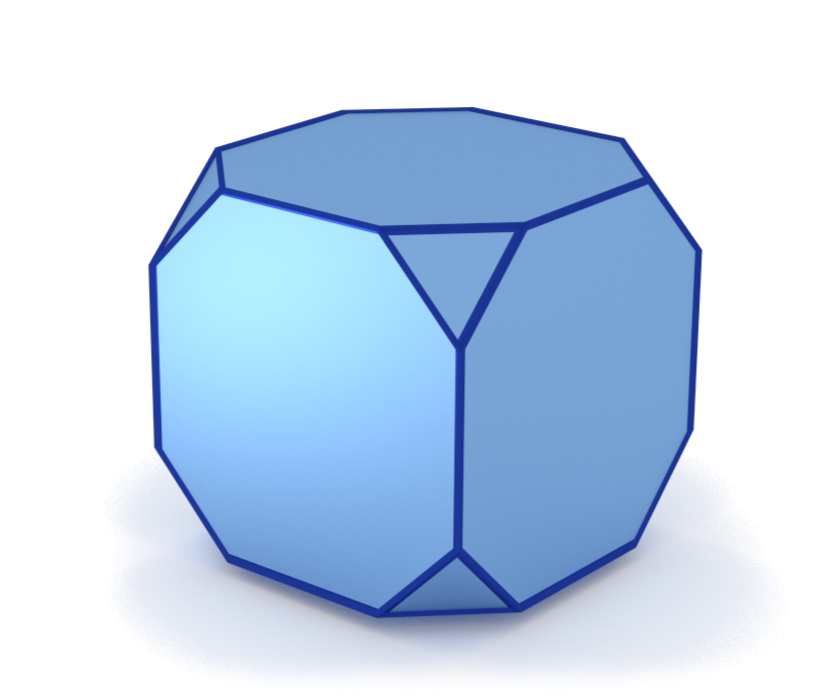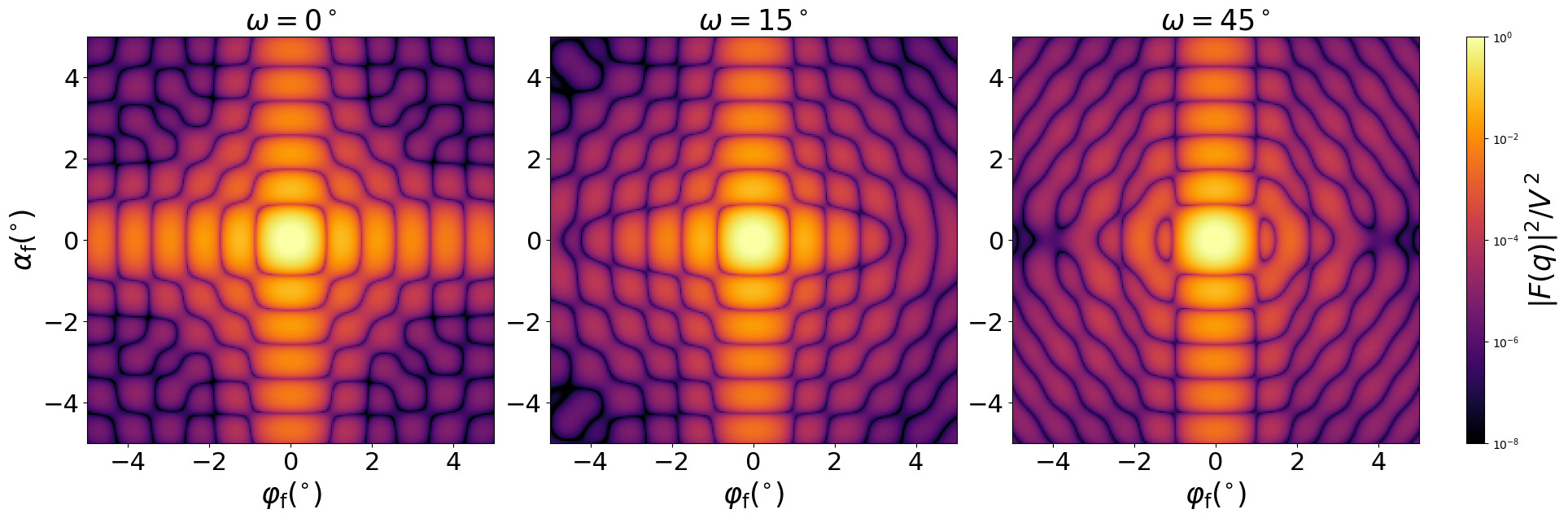### TruncatedCube

A cube whose eight vertices have been removed. The truncated part of each vertex is a trirectangular tetrahedron.#### Constructor

TruncatedCube(L, t)


Parameters:

• L, length of the full cube
• t, side length of the trirectangular tetrahedron removed from the cube’s vertices

Constraint:

$t \le L/2$

#### Usage

As for any other Form factor.

#### Implementation

Class TruncatedCube inherits from the interface class IFormFactor .

Form factor computation is based on the generic form factor of a polyhedron provided by libformfactor .

Volume has been validated against $$V= L^3 - \dfrac{4}{3} t^3.$$

#### Example

Scattering by uncorrelated, oriented truncated cubes for horizontal incidence. Rotation around $z$ axis:Generated by Examples/ff/TruncatedCube.py .

#### History

Reimplemented in BornAgain 1.6 using the generic polyhedron form factor [Wuttke 2021]. Motivated by [Croset 2017].